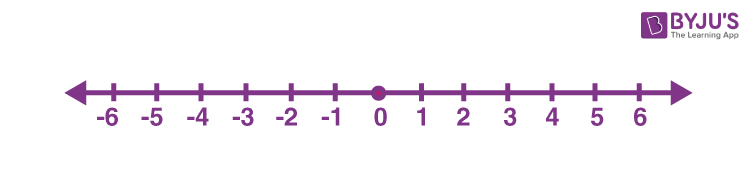# Ordering of Integers

Ordering of integers is stated for the series or a sequence where numbers are arranged in an order. The integers are ordered on a number line based on positive integers and negative integers. The integers that are greater than 0 are positive integers and integers less than 0 are negative integers.

As we know, the arrangement of numbers can be done in two ways:

In ascending order, the integers are arranged from smallest to largest value whereas in descending order the integers are arranged from largest to smallest value.

## Ordering of Integers on Number Line

As we have already learned, the integers are represented on a number line. The center of the number line is marked as 0. On the left side of 0, the negative integers are arranged in ascending order from left to right. On the right side of 0, the positive integers are arranged in ascending order from left to right. Thus, we can conclude that, on both sides of the 0, the integers are arranged in ascending order on the number line.In the above figure, we can see, the integers from -6 to -1, are arranged in ascending order from left to right and the integers from +1 to +6 are arranged in ascending order from left to right. So basically, all the integers from -6 to +6, are in ascending order from left to right whereas integers from right to left (+6 to -6) are in descending order.

Hence, we can say, -6 is smaller than all the integers marked on the number line (in the above figure) whereas 6 is the greatest of the integers.

Therefore, we can order the integers as:

-6 < -5 < -4 < -3 < -2 < -1 < 0 < 1 < 2 < 3 < 4 < 5 < 6

 Facts: Order of Integers on left side of the number line are smaller than 0 and order of integers on the right side of the number line are greater than 0 The more the integer is negative, the more its value is smaller The more the integer is positive, the more its value is greater Zero is neither a negative integer nor a positive integer All positive integers are greater than all the negative integers

## Comparing Integers

One integer can be either greater or smaller than another integer. Thus, to compare two integers we use symbols greater than (>) and less than (<). Also, if two integers are equal to each other then we use the ‘equal to’ (=) symbol. See the examples below:

• 0 > – 8
• 8 > – 5
• 0 < 3
• -1 > -10
• 10 < 12
• -20 < 1
• -100 < -10
• 100 > -200

From the above examples, we can observe that, the more an integer is negative, the less its value is.

## Solved Examples

Q.1: Which integers lie between 0 and -3?

Solution: By the order of integers, we know that,

-3 < -2 < -1 < 0

Therefore, there are two integers between 0 and -3, they are -1 and -2.

Q.2: Which is the largest integer and smallest integer among the following?

8, 10, -12, 0, 23, 1, 3, -7, -22

Solution: Given, the integers are:

8, 10, -12, 0, 23, 1, 3, -7, -22

Smallest integer = -22

Largest integer = 23

Q.3: Rama is at -2. In which direction and how many steps should he move to reach at – 6?

Solution: Rama has to move 4 steps to the left of -2 to reach -6.

Q.4: Which number will we reach if we move 5 steps to the right of –3?

Solution: If we move 5 steps to the right of -3 we reach 2.

### Practice Questions

1. Represent the following numbers on a number line: -1, 3, 0, -9, 8, -7
2. Do the ordering of the integers according to the number line. 8, 0, -9, 1, 3, 4, -2, -6, 7.
3. If we move 5 steps to the right of -5, where do we reach?
4. If the temperature is reduced to 15°C below 0°C, then how to represent it on the number line?

## Frequently Asked Questions on Ordering of Integers

### What are the two types of ordering integers?

The two types of ordering of integers are positive and negative integers. The integers that are greater than 0 are positive integers and integers less than 0 are negative integers.

### What are the four rules of integers?

Adding two integers results in an integer, having a sign of the greater number. Multiplication and division of two opposite sign integers results in negative integers and two same sign integers result in positive integers. Any integer multiplied by zero results in zero value and any integers multiplied by 1 results in the same value.

### How to compare and order integers?

Integers on the left side of 0 on the number line get smaller as we move from right to left whereas integers on the right side of 0 get greater as we move from left to right. Therefore, to compare two integers we can use greater than (>) or less than (

### How to order the integers from lowest to highest? Give an example.

The ordering of integers can be done from lowest to highest in ascending order. For example, if we integers such as, 5, -1, 0, 3, -4, -6, 1, 2, then the order of the given integers from lowest to highest will be:
-6

### Which is the smallest and largest integers among the orderer integers of -5 to 5?

If the integers are ordered from -5 to 5, then;
-5 < -4 < -3 < -2 < -1 < 0 < 1 < 2 < 3 < 4 < 5
Thus, from the above arrangement, we can say the smallest integer is -5 and greatest integer is 5